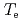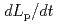We performed the disruption simulation using DINA code to investigate the effect of the electron temperatureon the plasma current decay after the initial phase of current quench (CQ). In this calculation, we used the measuredprofile during the initial phase of CQ. After the initial phase of CQ, we assumed that theprofile does not change in time and used the value at the end of the initial phase of current quench becauseprofile could not be measured after the initial phase of CQ. From the simulation results, it was found that the time evolution of plasma current calculated by DINA was similar to experimental one in this calculation. However, the time evolution ofprofile in this calculation was different from the measuredprofile because Te after first mini-collapse rapidly decreased until the value below a measurement limit (less than 0.1 keV). Moreover, the time evolution of poloidal cross-section S calculated by DINA code was rapidly decreased although the experimental one was gradually decreased. The plasma current decay during the disruption is determined by various parameters,,and S. It is necessary to evaluate the effect ofprofile on the plasma current decay after the initial phase of CQ by using various assumedmodel and DINA code.